# Theory of Graph

A graph says how the dependent quantity changes its value with respect to change in vol of independent quantity (variable/parameter).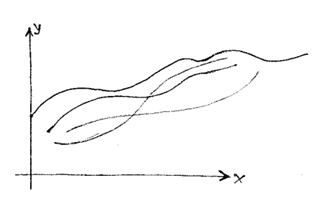Information that we obtain from the graph.

1. Pattern of dependency of one variable on other variable.
2. The nature of dependency, i.e. how a variable in changing with other variable.
3. The rate of change of one variable to other variable at any point.
4. The extreme values of dependent variable.
5. From a graph of a function, we can obtain domain, range, nature, period, etc. of the function.

Domain is the value on X-axis associated with graph.

Range is the value on Y-axis associated with graph.

Use of application of derivatives in Graph Theory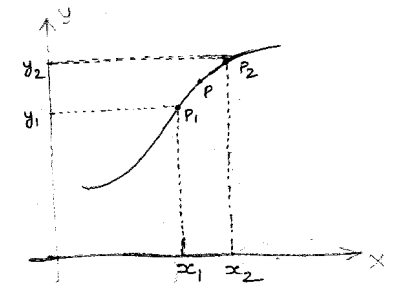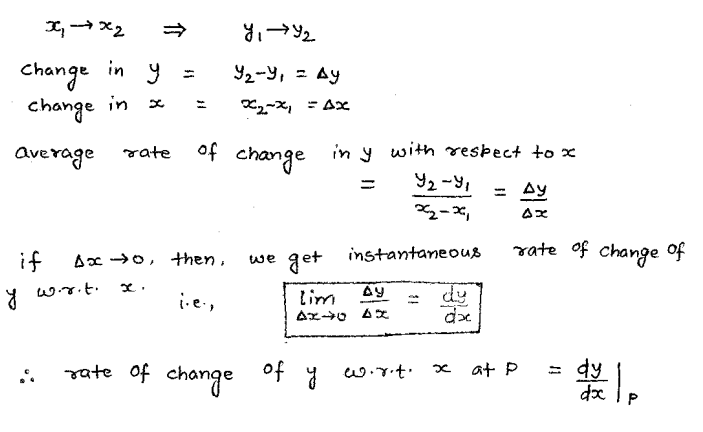Use of application of derivatives in Graph Theory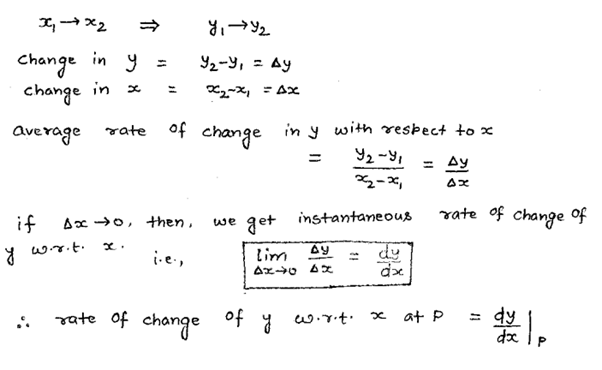Application of “rate of change” in Physics: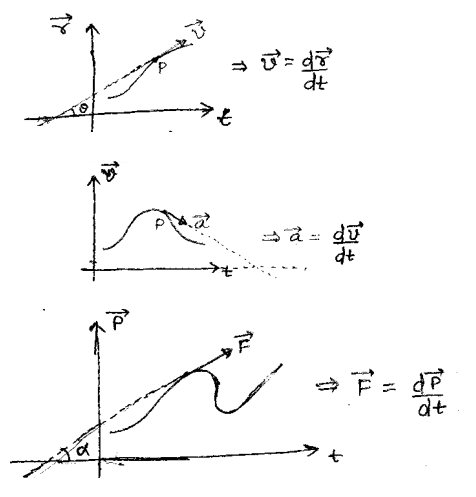To find the “slope of tangent” to the curve: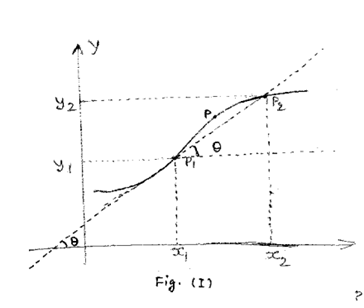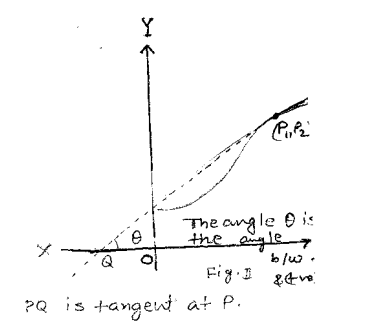Line joining two points on the curve is called chord.

If P1 & P2 become closer and closer together, then at a point P1, P1 & P2 will become adjacent point and then the chord will be known as tangent.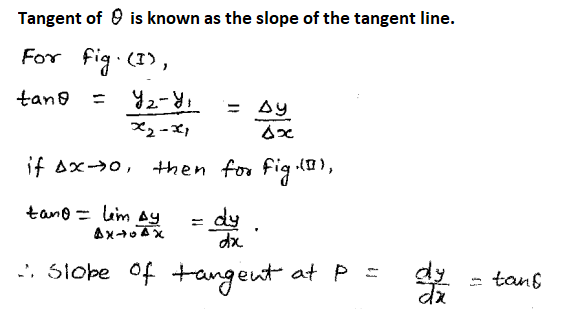Post By : Ramashankar Rajppot 25 Jan, 2020 2294 views Maths